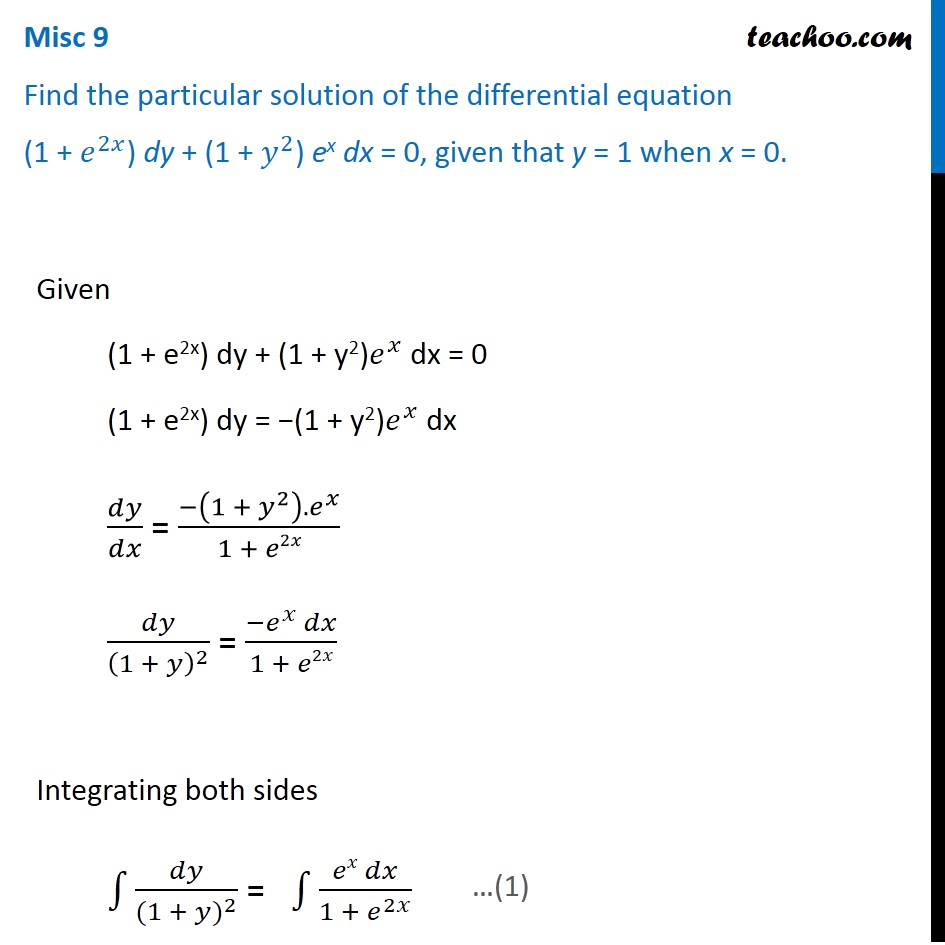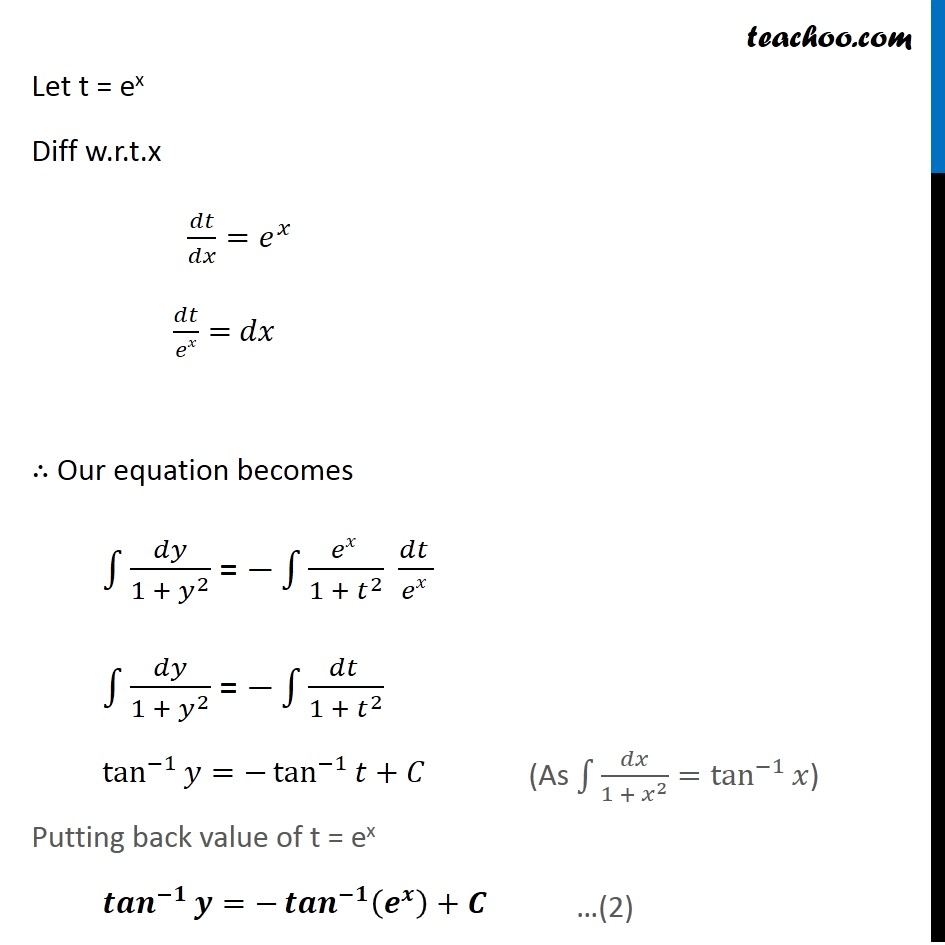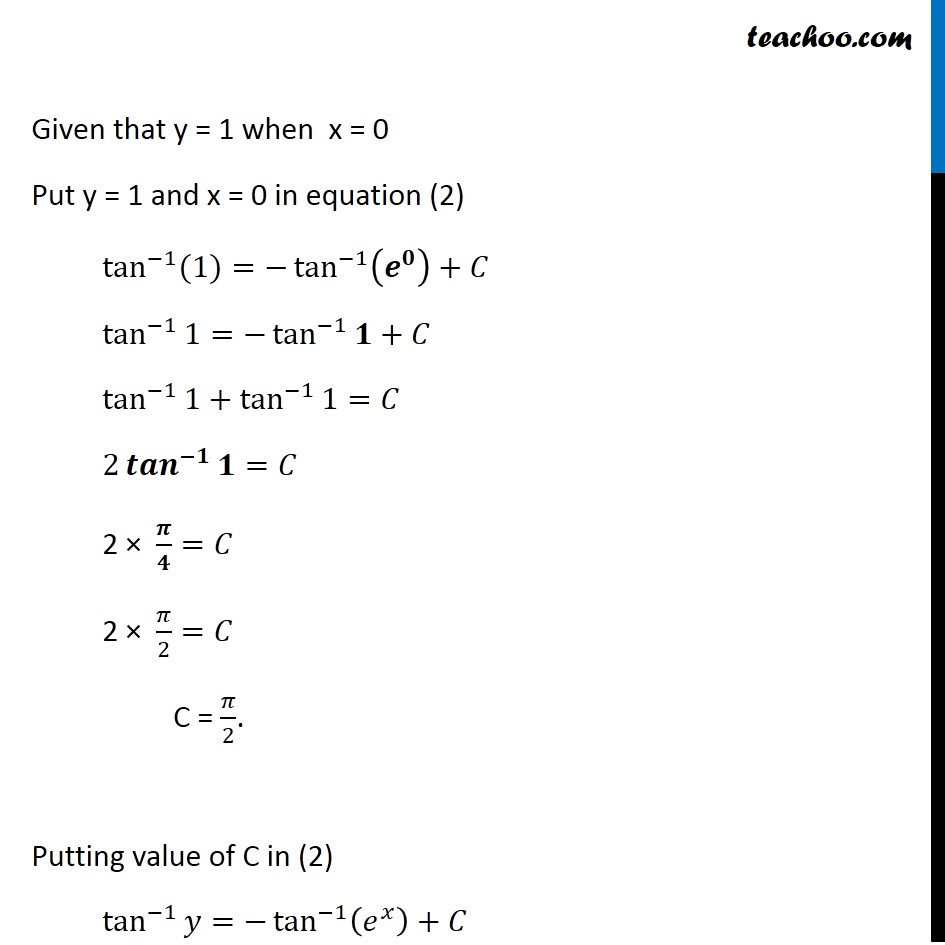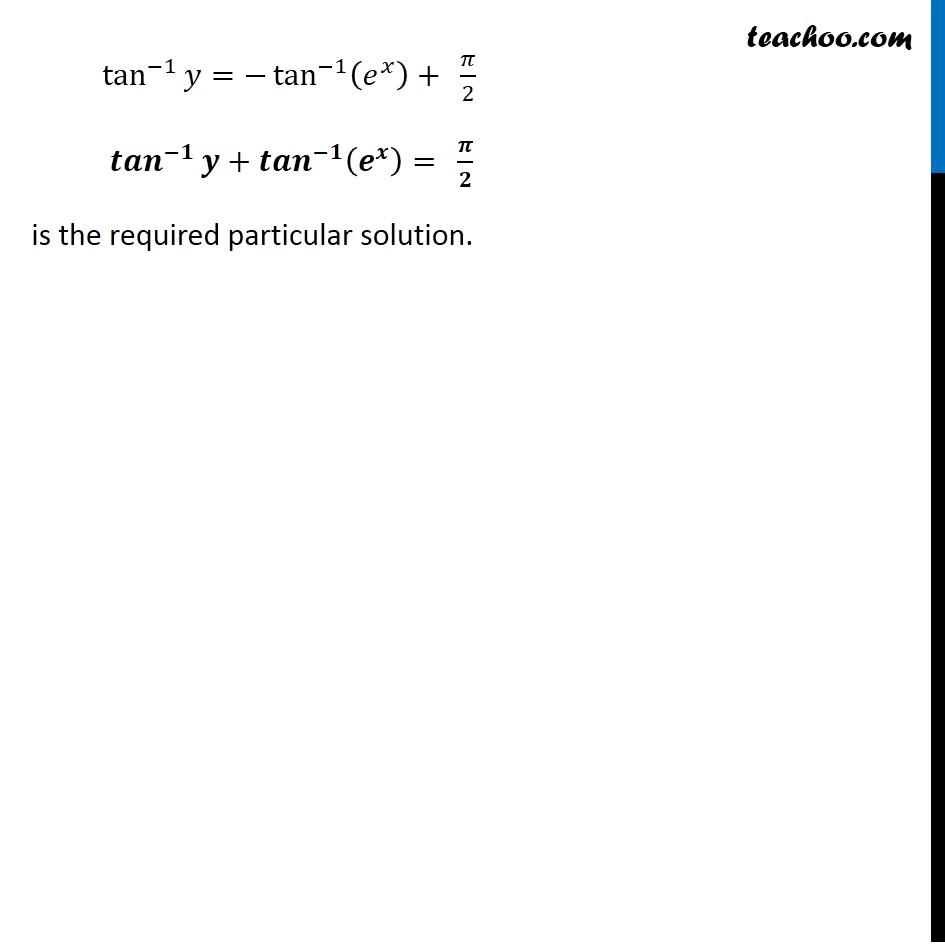Miscellaneous

Chapter 9 Class 12 Differential Equations
Serial order wiseLearn in your speed, with individual attention - Teachoo Maths 1-on-1 Class

### Transcript

Misc 7 Find the particular solution of the differential equation (1 + 𝑒^2𝑥) dy + (1 + 𝑦^2) ex dx = 0, given that y = 1 when x = 0. Given (1 + e2x) dy + (1 + y2)𝑒^𝑥 dx = 0 (1 + e2x) dy = −(1 + y2)𝑒^𝑥 dx 𝑑𝑦/𝑑𝑥 = (−(1 + 𝑦^2 ).𝑒^𝑥)/(1 + 𝑒2𝑥) 𝑑𝑦/(1 + 𝑦)^2 = (−𝑒^𝑥 𝑑𝑥)/(1 + 𝑒2𝑥) Integrating both sides ∫1▒𝑑𝑦/〖(1 + 𝑦)〗^2 = ∫1▒(𝑒𝑥 𝑑𝑥)/〖1 + 𝑒〗^2𝑥 …(1) Let t = ex Diff w.r.t.x 𝑑𝑡/𝑑𝑥=𝑒^𝑥 𝑑𝑡/𝑒𝑥= 𝑑𝑥 ∴ Our equation becomes ∫1▒𝑑𝑦/〖1 + 𝑦〗^2 = −∫1▒〖(𝑒𝑥 )/(1 + 𝑡^2 ) (𝑑𝑡 )/(𝑒𝑥 )〗 ∫1▒𝑑𝑦/〖1 + 𝑦〗^2 = −∫1▒〖(𝑑𝑡 )/(1 + 𝑡^2 ) 〗 tan^(−1)⁡𝑦=−tan^(−1)⁡𝑡+𝐶 Putting back value of t = ex 〖𝒕𝒂𝒏〗^(−𝟏)⁡𝒚=−〖𝒕𝒂𝒏〗^(−𝟏)⁡(𝒆^𝒙 )+𝑪 (As ∫1▒𝑑𝑥/〖1 + 𝑥〗^2 =tan^(−1)⁡𝑥) …(2) Given that y = 1 when x = 0 Put y = 1 and x = 0 in equation (2) tan^(−1)⁡〖(1)〗=−tan^(−1)⁡(𝒆^𝟎 )+𝐶 tan^(−1)⁡1=−tan^(−1)⁡𝟏+𝐶 tan^(−1)⁡1+tan^(−1)⁡1=𝐶 2 〖𝒕𝒂𝒏〗^(−𝟏)⁡𝟏=𝐶 2 × 𝝅/𝟒=𝐶 2 × 𝜋/2=𝐶 C = 𝜋/2. Putting value of C in (2) tan^(−1)⁡𝑦=−tan^(−1)⁡(𝑒^𝑥 )+𝐶 tan^(−1)⁡𝑦=−tan^(−1)⁡(𝑒^𝑥 )+" " 𝜋/2 〖𝒕𝒂𝒏〗^(−𝟏)⁡𝒚+〖𝒕𝒂𝒏〗^(−𝟏)⁡(𝒆^𝒙 )=" " 𝝅/𝟐 is the required particular solution.